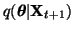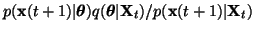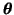Next: Acknowledgements Up: Discussion Previous: Independent and weakly coupled

## Future directions

In this work, adaptation of the approximation of the posterior density was done in batches, i.e., all the observations were processed before adaptation. It would be straight-forward to derive an on-line version of learning, however. For each new sample the new posterior approximationwould be adapted by minimising the misfit between the new approximation and. Heredenotes all the unknown variables of the model, including factors, parameters of the mappings and hyperparameters. This type of on-line learning would be essentially a version of Kalman filtering .

The nonlinear dynamic model used here is universal in the sense that in principle any data generating process can be modelled by it with any given precision. The significance of this property is mostly theoretical since in practice the amount of observations limit the complexity of models which can be reliably learned from the data. The generalisation ability of the models can be improved by prior knowledge about the model structure. This should also make it easier to identify the factors with physical quantities of the observed system.

Like the NLFA algorithm, the proposed NDFA algorithm scales quadratically as a function of the dimension of factor space and several times larger factor spaces than in the experiments reported here are thus computationally feasible. However, with high-dimensional nonlinear models the interpretation of the results can be rather difficult. Due to the approximation made in the posterior, the algorithm was shown to extract factors each of which can be identified with one of the underlying independent processes. This should happen more reliably if the prior model would also promote sparse temporal couplings between the underlying factors, e.g., by using a sparse prior on the weight matrices of the MLP networks. Use of non-Gaussian model for the innovation process m(t) should also aid at the interpretation of the results in a similar way as non-Gaussian model of factors in linear factor analysis.

Another example of using prior knowledge could be implementation of memories with specific structure. The state representation can in principle learn any memory, but learning complex structures can be difficult in practice. It is also possible that some of the states or control signals of the underlying dynamical process are directly measured and they can then be included in the model as known external inputs.Next: Acknowledgements Up: Discussion Previous: Independent and weakly coupled
Harri Valpola
2000-10-17